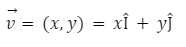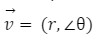• Call Now

1800-102-2727•

# Scalar and Vector: Representation, Definition, Examples

Have you heard two terms Distance and Displacement? Both are used for quantifying length traveled by some object. Distance can be represented by magnitude only (say 5 km); Displacement cannot, it needs a direction also.

## Types of Physical Quantities

Any physical quantity can be divided into three parts:

1. Scalar quantities
2. Vector quantities
3. Tensor quantities

## Scalar Quantities

• A physical quantity with only magnitude, such as time and temperature is known as scalar quantity.
• They do not have any particular direction.
• Some real life examples of scalar quantities are given below:
• Distance: Suppose you are at your home and want to go to a garden after your school(as shown in figure). So you have to travel 5 km for school and again 5 km for the garden. Hence the total distance traveled by you will be 10 km.• Density: Density is defined as mass per unit volume. Density is denoted by magnitude only. For example, density of water is 1000 kg/m3.

## Vector Quantities

• A physical quantity with both magnitude and direction is known as vector quantities.
• Some real life examples of vector quantities are given below:
• Displacement: Considering the above example of going home, school and garden.Then to calculate the displacement we need magnitude and direction. The magnitude of displacement will be smallest distance between home and garden, which is 50 km and the direction will be home to the garden.• Force: Let's lift a bucket of water, what is the force you have applied on the bucket. So, for defining the force on a bucket we need magnitude and direction. Magnitude of force will be equal to the weight of the bucket and water, and direction will be upward.## Tensor Quantities

Quantities with magnitude as well as direction but don't obey the laws of vector addition or subtraction are tensor quantities. For example- stress, strain, electric current,etc.

Tensors are generalization of scalar and vector, scalar is zero order tensors, vector is first order tensor.

## Difference Between Scalar and Vector Quantities## Representation of Vector Quantities

For the representation of vector quantities we use arrows, the length of the arrow represents the magnitude of vector quantities and arrow head represents the direction of the vector.Let's consider an arrow as shown in figure above is representing a physical quantity having the length 2 units and is headed toward the east. So the magnitude of the quantity is 2 units and the direction is toward the east.

Symbolically, Vector is denoted by a right arrow over the letters representing the tail and head of the vector respectively.

## Notation of Vector• Rectangular notation

In Rectangular notation, the vector is located in a rectangular coordinate system, as shown in above figure .

The rectangular notation for vector is• Polar notation

In the polar notation, we denote the vector magnitude by r,

Where r ≥ 0, and its angle θ with the positive x- axis as shown in the figure.

The Polar notation for vector is## Practice Problems of Scalar and Vector

Question 1. Which of the following quantities is scalar ?

(a)  Displacement (b) Acceleration (c) Temperature (d) Force

Answer: To define Temperature only magnitude is needed. So, temperature is a scalar quantity. Hence option (c) is correct.

Question 2. Which of the following quantities is vector ?

(a) Speed (b) Velocity (c) Mass (d) Distance

Answer: To define Velocity magnitude and direction are needed.so, velocity is a vector quantity. Hence option (b) is correct.

Question 3. What is the magnitude of the quantity represented by the vector AB shown in figure below?(a) 3 units (b) 4 units (c) 5 units (d) 6 units

Answer: The magnitude of the vector will be 5 units. Hence option (c) is correct.

Question 4. What is the direction of the quantity represented by the vector shown in figure below?(a) East (b) North (c) North-East (d) North-South

## FAQs of Scalar and Vector

Question 1. What is the scalar quantity ?

Answer: The quantity which is described only by its magnitude is called scalar quantity .

Question 2. What is vector quantity?

Answer: The quantity which is described by both its magnitude and direction is called vector quantity.

Question 3. Speed is scalar quantity or vector quantity?

Answer: speed is described by magnitude only for ex. 5 m/s, so scalar quantity.

Question 4. Force is scalar quantity or vector quantity?

Answer: Force is described by both magnitude as well as direction ,so vector quantity.

## NCERT Class 11 Physics Chapters

 Physical World Units and Measurements Motion in a Straight Line Motion in a Plane Laws of Motion Work Energy and Power Particles and Rotational Motion Gravitation Mechanical Properties of Solids Mechanical Properties in Liquids Thermal Properties of Matter Thermodynamics Kinetic Theory Oscillations WavesTalk to our expert
Resend OTP Timer =
By submitting up, I agree to receive all the Whatsapp communication on my registered number and Aakash terms and conditions and privacy policy